#Gauge invariance and unitarity

+ 2 like - 0 dislike
1363 views

Suppose for simplicity we have an abelian gauge theory. We want to quantize it in terms of 4-potential $A_{\mu}$.

If we impose the covariant gauge (like $\partial_{\mu}A^{\mu} = 0$) on the physical state $|\Psi\rangle$, namely

$$\langle\Psi_{1}|\partial_{\mu}A^{\mu}|\Psi_{2}\rangle = 0,$$

then it turns out that the physical state contains an equal number of time-like and longitudinal photons; they are associated with creation-destruction operators attached to $A_{0}, \mathbf{A}(p)\cdot\mathbf p$ respectively, and the former (time-like) has negative norm. But the latter (longitudinal) provides that the norm of the total state, with equal number of time-like and longitudinal photons, is zero. In the literature this procedure of the quantization is called the Gupta-Bleuler quantization.

In this gauge the relation between unitarity and gauge invariance is thus obvious: by breaking the gauge invariance we thus break the unitarity, since the negative norm states enter the game.

Suppose now we want to quantize the theory in a gauge $A_{0}= 0$. There is a remaining set of time independent gauge transformations related to longitudinal degrees of freedom, for which the Gauss constraint
$$G(x) = \nabla \cdot \mathbf{E} - \rho$$
is a Noether current. Precisely, the hamiltonian $H$ of the theory commutes with $G$. To eliminate such degrees from the physical state, we have to require
$$\tag 1 G(x)|\Psi\rangle = 0$$
The breaking of gauge invariance in general means that $(1)$ is violated with time.

My question is following. In the gauge $A_{0} = 0$ I don't see why the presence of longitudinal photons causes the violation of the unitarity in this gauge. Precisely, in this gauge the situation is similar to the massive QED, where longitudinal degree of freedom is present, as I think. Could you clarify where the unitarity violation is hidden in the $A_{0}=0$ gauge?

edited Dec 27, 2016

When fields are coupled and solutions are not known (or evident), the only way to make sure the unitarity holds is to give purely gauge increments to the fields and see that their contributions to any physical S-matrix element is zero (or disappears). It is done in the Lagrangian formulation.

Unitarity of the theory leads to the relation between scattering amplitudes and cross sections, given by the optical theorem. In order for the optical theorem to hold, the numerator of the propagator needs to be a sum over all physical spin states. For example, for massive spin-1 field in the numerator we have a sum over all three physical states and the optical theorem holds. However, for massless spin-1 field the numerator of the propagator is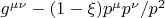, which is not the sum over physical states: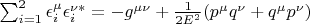, where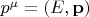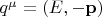. From the fact that the propagator is gauge invariant and that Ward identity holds for the cross-section, all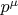terms disappear and the optical theorem holds.That is why gauge invariance is crucial for unitarity.
It is important to understand, however, that gauge invariance is not observable and is not even a symmetry. It is just convenient to build local Lagrangians. It has its drawbacks. For example, to calculate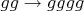process at tree level in QCD one needs around 100 pages of calculations, whereas the final result can be written compactly in one single line (see Parke-Taylor formula), using spinor-helicity formalism. In other methods, such as BCFW recursion relations, no one even builds local Lagrangians, and in the Amplituhedron approach there is no spacetime at all.
 Please use answers only to (at least partly) answer questions. To comment, discuss, or ask for clarification, leave a comment instead. To mask links under text, please type your text, highlight it, and click the "link" button. You can then enter your link URL. Please consult the FAQ for as to how to format your post. This is the answer box; if you want to write a comment instead, please use the 'add comment' button. Live preview (may slow down editor)   Preview Your name to display (optional): Email me at this address if my answer is selected or commented on: Privacy: Your email address will only be used for sending these notifications. Anti-spam verification: If you are a human please identify the position of the character covered by the symbol $\varnothing$ in the following word:p$\hbar$ysic$\varnothing$OverflowThen drag the red bullet below over the corresponding character of our banner. When you drop it there, the bullet changes to green (on slow internet connections after a few seconds). To avoid this verification in future, please log in or register.